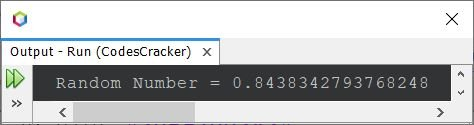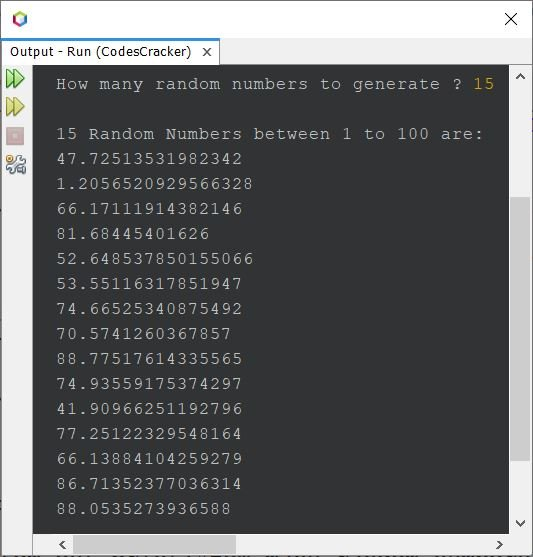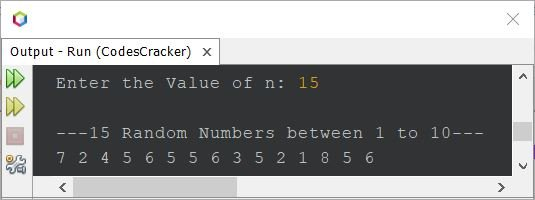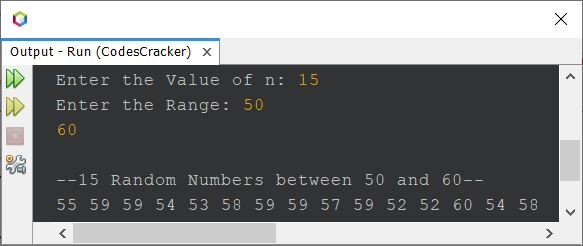# Java Program to Generate Random Numbers

• Generate and print a random number in Java using Math.random()
• Generate and print n random numbers in Java
• Generate and print n random numbers between 1 to 100 in Java
• Generate and print n random numbers between given range in Java
• Generate and print random numbers in Java using Random class

## Generate and Print a Random Number in Java

The question is, write a Java program to generate a random number. The program given below is its answer:

```import java.lang.Math;

public class CodesCracker
{
public static void main(String[] args)
{
double num = Math.random();
System.out.println("Random Number = " +num);
}
}```

The snapshot given below shows the sample output produced by above Java program:Every time you execute the above program, there would be a different random number generated.

Note - Since the random number returned by Math.random() is of double type, therefore I've stored the returned random number in a variable of double type. You can use other like float, but you need to typecast, to avoid loosy conversion from double to float.

## Generate and Print n Random Numbers in Java

Since previous program generate and prints only one random number at a time. Therefore, let's allow user to define the amount of random numbers to generate, then print, using the program given below:

```import java.util.Scanner;
import java.lang.Math;

public class CodesCracker
{
public static void main(String[] args)
{
Scanner scan = new Scanner(System.in);

System.out.print("How many random numbers to generate ? ");
int n = scan.nextInt();

System.out.println("\nList of " +n+ " Random Numbers:");
for(int i=0; i<n; i++)
System.out.println(Math.random());
}
}```

The sample run with user input 7 is shown in the snapshot given below:## Generate Random Numbers between 1 to 100 in Java

If you've not noticed, then let me tell you, that the Math.random() are generating random numbers between 0 to 1. Therefore to generate random numbers between 1 to 100, we need to use the program given below.

```import java.util.Scanner;
import java.lang.Math;

public class CodesCracker
{
public static void main(String[] args)
{
Scanner scan = new Scanner(System.in);

System.out.print("How many random numbers to generate ? ");
int n = scan.nextInt();

System.out.println("\n" +n+ " Random Numbers between 1 to 100 are:");
for(int i=0; i<n; i++)
{
double num = Math.random()*(100);
System.out.println(num);
}
}
}```

The sample run of above program with user input 15 is shown in the snapshot given below:### Generate Random Numbers between 1 to 10 - Whole Number

Since previous program generates random numbers that are of double type. Therefore to generate random numbers of int type, a whole number, then use following program:

```import java.util.Scanner;
import java.lang.Math;

public class CodesCracker
{
public static void main(String[] args)
{
Scanner scan = new Scanner(System.in);

System.out.print("Enter the Value of n: ");
int n = scan.nextInt();

System.out.println("\n---" +n+ " Random Numbers between 1 to 10---");
for(int i=0; i<n; i++)
{
double num = Math.random()*(10);
int wholeNum = (int)num;
System.out.print(wholeNum+ " ");
}
}
}```

Here is its sample run with same user input as of previous program:## Generate Random Numbers between Given Range in Java

This program generates n random numbers between given range. The value of n and the range are all received by user at run-time of the program:

```import java.util.Scanner;
import java.lang.Math;

public class CodesCracker
{
public static void main(String[] args)
{
Scanner scan = new Scanner(System.in);

System.out.print("Enter the Value of n: ");
int n = scan.nextInt();

System.out.print("Enter the Range: ");
int start = scan.nextInt();
int end = scan.nextInt();

System.out.println("\n--"+n+" Random Numbers between "+start+" and "+end+"--");
for(int i=0; i<n; i++)
{
double num = Math.random()*(end-start+1)+start;
int wholeNum = (int)num;
System.out.print(wholeNum+ " ");
}
}
}```

The snapshot given below shows the sample run of above program, with user input 15 as size, 50 as starting number and 60 as ending number, to generate 15 random numbers between 50 to 60:## Generate Random Numbers in Java using Random Class

This is the last program of this article, created using Random class to generate random numbers, instead of Math.random()

```import java.util.Random;

public class CodesCracker
{
public static void main(String[] args)
{
Random r = new Random();
System.out.println("10 Random Numbers from 0 to 4");
for(int i=0; i<10; i++)
System.out.println(r.nextInt(5));
}
}```

The output produced by above Java program is:

```10 Random Numbers from 0 to 4
3
3
1
2
3
4
4
1
0
1```

#### Same Program in Other Languages

Java Online Test

« Previous Program Next Program »